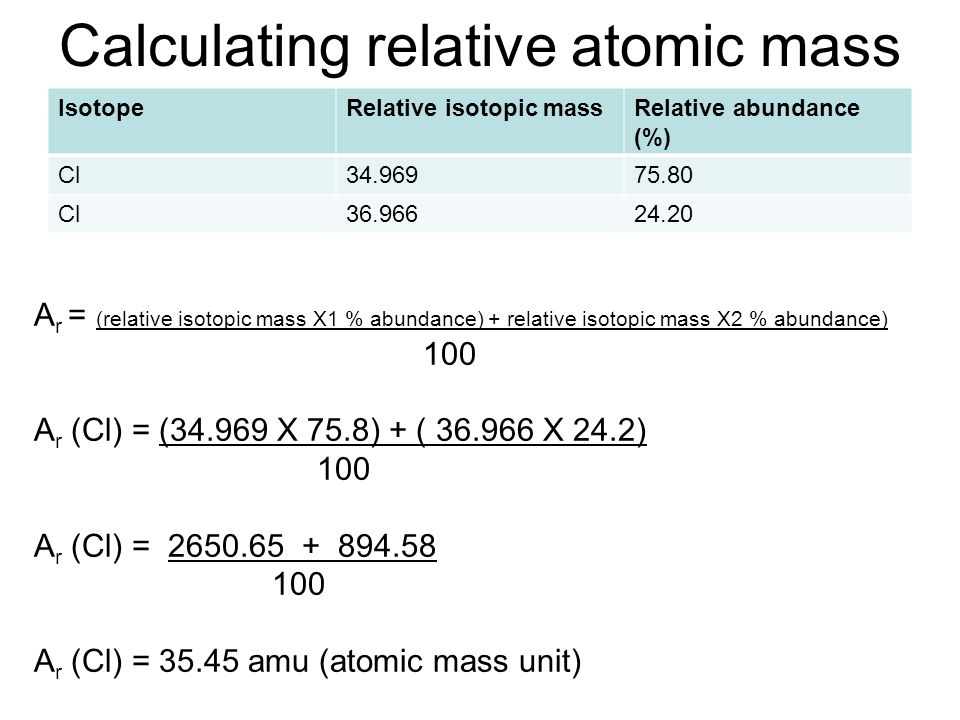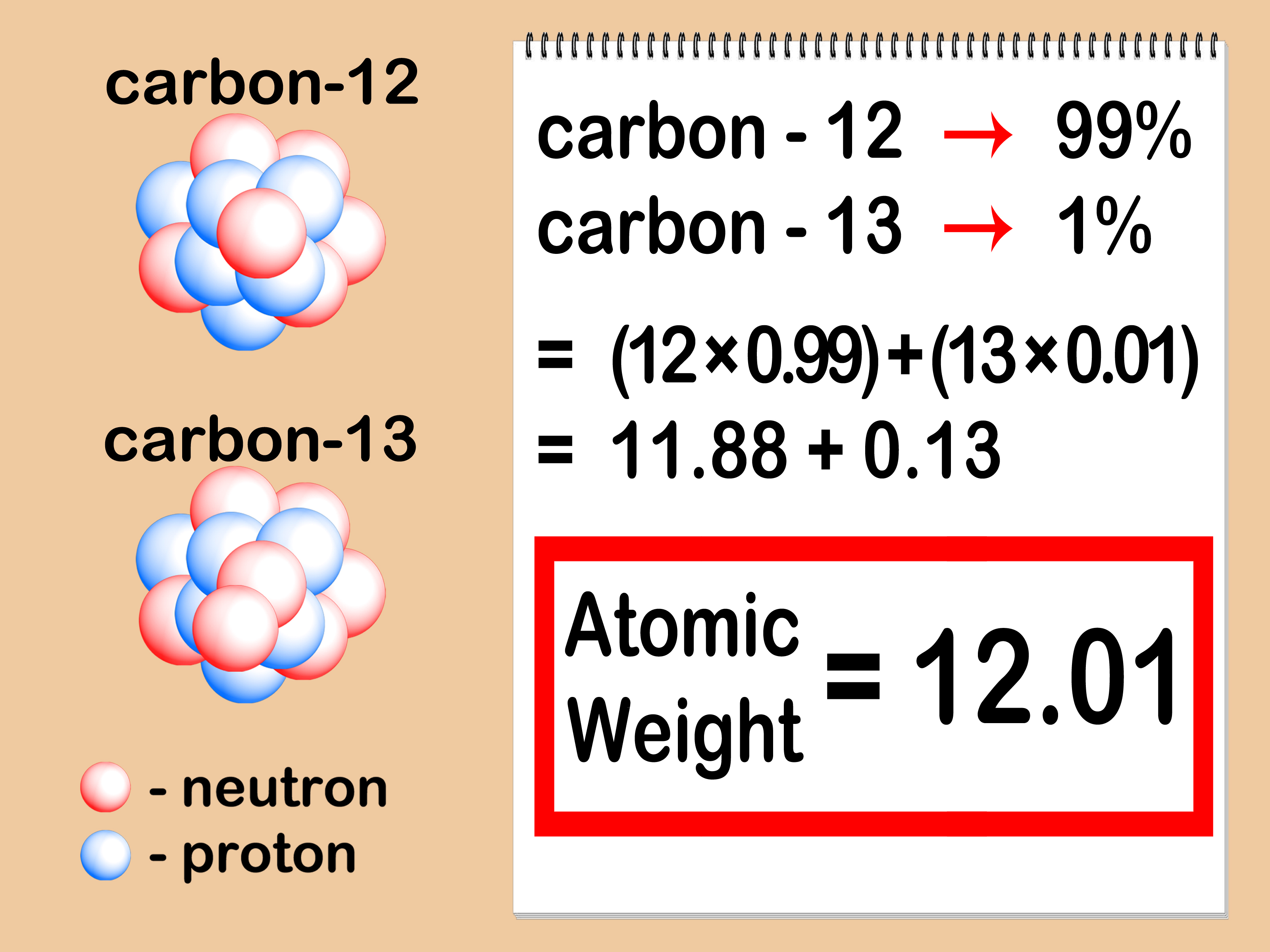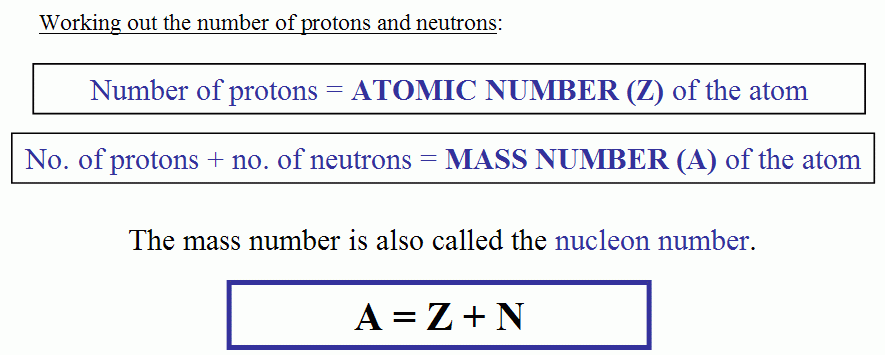# How To Find Atomic Mass & Number Of Elements

The basic units of matter are atoms. They are the tiniest component of an element. It is the simplest form of a substance that cannot be broken down. They have various specific characteristics that determine their physical and chemical nature.

Atomic mass one of those properties. In this article, we will discuss atomic mass and its role in chemistry. The mass of an atom of an element is called the atomic mass. It is the sum of electron, neutron, and proton.

### How To Calculate Atomic Mass of Isotopes

The variant of a chemical element that has a different number of the neutron is called and IsotopeThe formula for calculating the atomic mass of an Isotope is given in the picture below:#### What is the Formula for Calculating Atomic Mass?

Atomic mass = Number of protons + number of neutrons + number of electrons

Mass number = A= Number of neutron + Number of proton + Number of electron#### What is the Formula For Atomic Mass?

Atomic mass is the sum total of masses of the individual particle of an atom that have three components:

• protons(positively charged particles)
• neutrons (non-charged particles)
• Electrons (negatively charged particles).

#### How Do You find Atomic Mass Without Neutrons?

You can also find the atomic mass of an element without knowing the number of the neutron by the method depicted in the picture below;#### Which Unit is Used for Measuring Atomic Mass

Unified atomic mass unit or dalton unit is used for measuring the Atomic mass